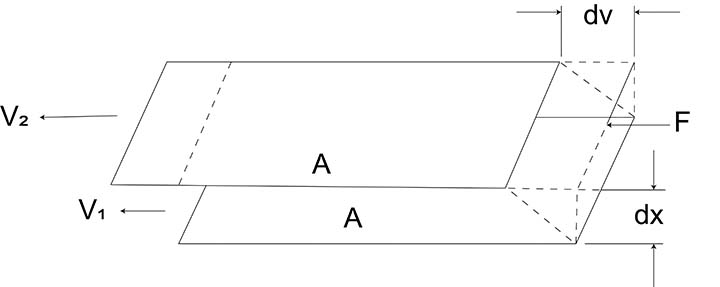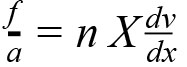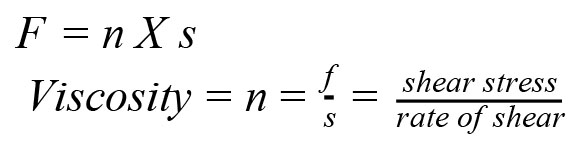The viscosity of a fluid is the property that tends to resist a shearing force. It can be thought of as the internal friction resulting when one layer of fluid is made to move in relation to another layer. A detailed discussion on viscosity is a major undertaking and this article serves to provide a basic understanding of viscosity and how it impacts on pumping.

Consider the model shown in Figure 1, which was used by Isaac Newton in first defining viscosity. It shows two parallel planes of fluid of area A separated, by a distance dx and moving in the same direction at different velocities V1 and V2.Figure 1.

The velocity distribution will be linear over the distance dx, and experiments show that the velocity gradient, dv dx is directly proportional to the force per unit area, fa .Where n is constant for a given liquid and is called its viscosity.

The velocity gradient, dv dx describes the shearing experienced by the intermediate layers as they move with respect to each other.

Therefore, it can be called the “rate of shear”, S. Also, the force per unit area fa can be simplified and called the “shear force” or “shear stress,” F. With these simplified terms, viscosity can be defined as follows:Article courtesy of Kelair Pumps Australia “When Pump Knowledge Matters”. Phone: 1300 789 466 or visit www.kelairpumps.com.au.

Related articles